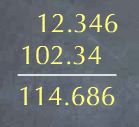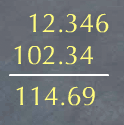# Stoichiometry Tutorials: Significant Figures

(from a complete OLI stoichiometry course)

In many of the problems in these tutorials, you will be asked to report your answer with a specific number of significant figures. This section provides a brief review of the topic. For a thorough review of significant figures, please see this web site.

Here are some examples of how to count significant figures.

Number Number of significant figures Explanation
12.3 (1.23 x 101) 3 Nonzero digits are always significant.
100.2 (1.002 x 102) 4 Zeros between non-zero digits are always significant.
0.093 (9.3 x 10-2) 2 Leading zeros (zeros before the first non-zero digit) are not significant.
120.30 (1.2030 x 102) 5 Trailing zeros after a decimal point are always significant.
4320 Ambiguous A trailing zero before the decimal point is ambiguous. To avoid this ambiguity, use scientific notation to indicate whether you mean 3 significant figures (4.32 x 103) or 4 significant figures (4.320 x 103).

## Significant figures from multiplication or division:

When multiplying or dividing, the number of significant figures in the result is equal to the smallest number of significant figures in one of the operands. For instance, given the following:

(1.74 * 4.3)/3.42

We first use our calculator to evaluate the expression:

(1.74 * 4.3)/3.42 = 2.187719 (from calculator).

The operand with the smallest number of significant figures is 4.3, so our answer should have 2 significant figures. We therefore round 2.187719 to 2.2 to get:

(1.74 * 4.3)/3.42 = 2.2 (to correct number of significant figures).

## Significant figures from addition or subtraction:

When adding or subtracting, the number of decimal places in the answer is equal to the operand with the smallest number of decimal places.

Consider 12.346 + 102.34. If we use a calculator to evaluate this sum, we get:The number of decimal places in our answer should be two, since the operand with the least decimal places is 102.34, and this has two decimal places. We should therefore round the result to two decimal places.## Questions:

The content above has been converted from Adobe Flash Player and may not display correctly.